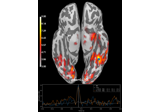# mne.viz.plot_events¶

mne.viz.plot_events(events, sfreq=None, first_samp=0, color=None, event_id=None, axes=None, equal_spacing=True, show=True)[source]

Plot events to get a visual display of the paradigm.

Parameters
eventsarray, shape (n_events, 3)

The events.

sfreq

The sample frequency. If None, data will be displayed in samples (not seconds).

first_sampint

The index of the first sample. Recordings made on Neuromag systems number samples relative to the system start (not relative to the beginning of the recording). In such cases the raw.first_samp attribute can be passed here. Default is 0.

color

Dictionary of event_id integers as keys and colors as values. If None, colors are automatically drawn from a default list (cycled through if number of events longer than list of default colors). Color can be any valid matplotlib color.

event_id

Dictionary of event labels (e.g. ‘aud_l’) as keys and their associated event_id values. Labels are used to plot a legend. If None, no legend is drawn.

axesinstance of Axes

The subplot handle.

equal_spacingbool

Use equal spacing between events in y-axis.

showbool

Show figure if True.

Returns
figmatplotlib.figure.Figure

The figure object containing the plot.

Notes

New in version 0.9.0.

## Examples using mne.viz.plot_events¶